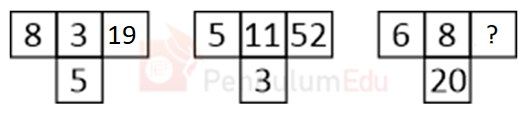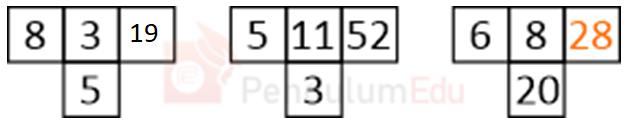Question of The Day17-06-2021

Choose the alternative that will replace “?” question markCorrect Answer : a ) 28

Explanation :

According to the question

The common logic applied here is

⇒ 8 * 3 = 24

⇒24 – 5 = 19

Similarly

⇒ 5 * 11 = 55

⇒ 55 – 3 = 52

Similarly

⇒ 6 * 8 = 48

⇒ 48 – 20 = 28Thus, 28 will replace “?”

Hence, (a) is the correct answer.0